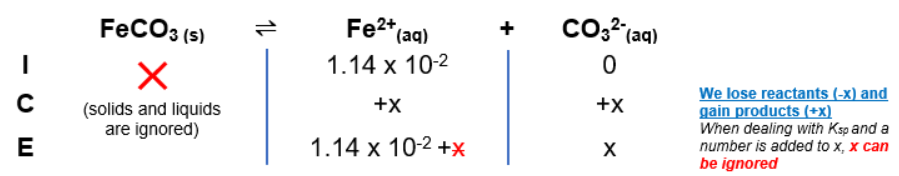# Problem: A solution of Na2CO3 is added dropwise to a solution that contains 1.14 x 10-2 M Fe2+ and 1.50 x 10-2 M Cd2+.What will be the concentration of Cd2+ be when Fe2+ begins to precipitate? Express the molar concentrations numerically.

###### FREE Expert Solution

To calculate the concentration of Cd2+ when Fe2+ starts to precipitate:

CO32- from soluble Na2CO3 will react with ions in solution to form precipitates:

Fe2+(aq) + CO32-(aq) ⇌ FeCO3(s) → Ksp =  2.10 x 10-11

•  higher Ksp → more soluble → precipitates last

Cd2+(aq) + CO32-(aq)  ⇌ CdCO3(s) → Ksp =  1.80 x 10-14

We will construct an ICE Chart to determine the concentration of CO32- needed to start precipitation of FeCO3The Ksp expression for FeCO3 is:

$\overline{){{\mathbf{K}}}_{{\mathbf{sp}}}{\mathbf{=}}\frac{\left[\mathrm{products}\right]}{\overline{)\left[\mathrm{reactants}\right]}}\mathbf{=}\mathbf{\left[}{\mathbf{Fe}}^{\mathbf{2}\mathbf{+}}\mathbf{\right]}\mathbf{\left[}{{\mathbf{CO}}_{\mathbf{3}}}^{\mathbf{2}\mathbf{-}}\mathbf{\right]}}$

**Solids are ignored in the Ksp expression

Solve for x

${\mathbf{K}}_{\mathbf{sp}}\mathbf{=}\mathbf{\left[}{\mathbf{Fe}}^{\mathbf{2}\mathbf{+}}\mathbf{\right]}\mathbf{\left[}{{\mathbf{CO}}_{\mathbf{3}}}^{\mathbf{2}\mathbf{-}}\mathbf{\right]}$

x = [CO32-] = 1.479 x 10-9 M CO32-

95% (197 ratings)###### Problem Details

A solution of Na2CO3 is added dropwise to a solution that contains 1.14 x 10-2 M Fe2+ and 1.50 x 10-2 M Cd2+.

What will be the concentration of Cd2+ be when Fe2+ begins to precipitate? Express the molar concentrations numerically.